﻿ 碎纸片拼接复原模型

# 碎纸片拼接复原模型Scraps of Paper Splicing Recovery Model

Abstract:
The main purpose of this paper is to explore how the paper scraps of neat edges could be restored. First, we can obtain the paper scraps of neat edges after cross cut and then length cut. With the help of the image-processing software, Matlab, we can transfer the images into corresponding matrix, and then binarize the matrix and get the 0-1 matrix. We classify the paper scraps with the same location features using cluster analysis. Then we match the first column and the last column in certain pixel matrix with the corresponding columns in other pixel matrix based on the edge matching. According to the edge matching, we determine the order of the pictures, splice them and then check them with necessary manual adjustment, so we get the transverse paper. Finally, the same method can be applied to the transverse paper while we match the lines instead of the columns and then we get the restored paper after manual adjustment.

1. 引言

2. 模型假设及符号说明

2.1. 模型假设

1) 设碎纸图片与真实文件纸张大小、颜色、边缘情况相同；

2) 假设碎纸照片边缘完整，不存在破损；

3) 假设所有碎纸片的扫描情况相同；

2.2. 符号说明

${A}_{i}$ ——编号i的图片的灰度矩阵；

${B}_{i}$ ——编号i的图片二值化处理后矩阵；

${C}_{i}$ ——编号i的图片二维边缘矩阵；

$D,{D}^{\prime },{D}^{″},{D}^{‴}$ ——边缘匹配度矩阵；

${E}_{i}$ ——编号 $i$ 的图片再次处理后的0-1矩阵；

$F$ ——边缘匹配度之和矩阵。

3. 问题分析

3.1. 中文碎纸片的分析

${A}_{i}=\left(\begin{array}{ccc}255& 255& 0\\ 255& 220& 150\\ 255& 0& 0\end{array}\right)$

${B}_{i}=\left(\begin{array}{ccc}0& 0& 1\\ 0& 1& 1\\ 0& 1& 1\end{array}\right)$

${D}_{ij}=\frac{元素相同的数量}{180}$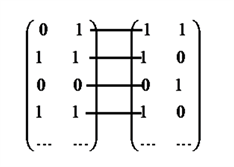Figure 1. The matching diagram of 014 and 128 two images

1) 搜索每一张碎纸片转化后二值化矩阵 ${C}_{i}$ 的每一行，若矩阵该行中存在数值1，则将该行全部赋值为1，若这一行元素全为0，则将该行全部赋值为0，其中1表示本行存在灰度小于255的像素，0表示不存在灰度小于255的像素，这样将209张碎纸片做出新的二值化矩阵 ${E}_{i}$

2) 同3.1的分析取边缘做边缘匹配得修改后的边缘匹配度矩阵 $D$ ，匹配度高则说明碎纸片的文字信息处于同一水平位置，见图2

3) 在2)完成后如果未完全匹配，则进行再人工干预，得到较优的结果图3

4) 得到很多组有相同位置的碎纸片后，在每一组内采用边缘匹配方法，这里为了防止出现两白边匹配造成碎纸片连接混乱的现象，要加以限制。方法为：若在组内做边缘匹配出现匹配度为1的情况，则暂时不连接此碎纸片，从剩余的碎纸片出发做边缘匹配与其他碎纸片连接，直到组内所有碎纸片均已覆盖。

5) 这样再通过一定人工干预可以得到拼接复原后的11横行碎纸片，再同样使用边缘匹配方法，将得到的11行碎纸条长边进行边缘匹配做出 $11×11$ 的匹配度矩阵后找最大匹配度作为连接的碎纸条，同样为了防止出现两白边匹配造成碎纸片连接混乱的现象，要加以限制。方法为：若在组内做边缘匹配出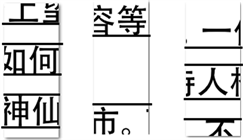Figure 2. The images after secondary handling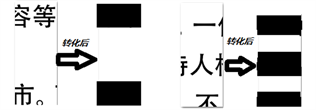Figure 3. The images after secondary handling

3.2. 英文碎纸片的分析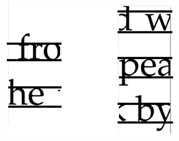Figure 4. Demonstrates picture 1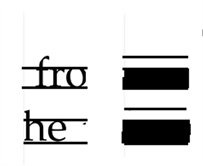Figure 5. Demonstrates picture 2

4. 模型的建立与求解

4.1. 中文碎纸片复原的模型建立与求解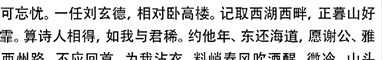Figure 6. The second set of splice results (1)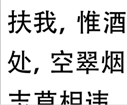Figure 7. The second set of splice results (2)

4.2. 英文碎纸片复原的模型建立与求解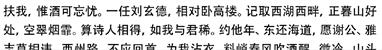Figure 8. The second set of results after splicing human intervention

4.3. 实现算法Table 3. Long-edge stitching result of shredded paperTable 4. The recovery results of Annex 3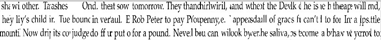Figure 9. Part of the mosaic picture of the results of the English group after completion

${G}_{x}=\left(\begin{array}{ccc}-1& 0& +1\\ -2& 0& +2\\ -1& 0& +1\end{array}\right)\ast A$ ${G}_{y}=\left(\begin{array}{ccc}+1& +2& +1\\ 0& 0& 0\\ -1& -2& -1\end{array}\right)\ast A$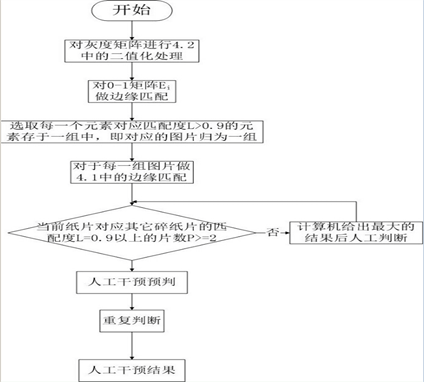Figure 10. The flow chartTable 5. The recovery results of Annex 4

$G=\sqrt{{G}_{x}^{2}+{G}_{y}^{2}}$

$\Theta =\mathrm{arctan}\left(\frac{{G}_{y}}{{G}_{x}}\right)$

4.4. 聚类精度和算法时间复杂度分析

1) 聚类精度分析

2) 时间复杂度分析

5. 模型的评价与推广

5.1. 模型的优点

5.2. 模型的缺点

5.3. 模型的推广

 周丰 (2007) 图像碎片自动拼接技术研究. 硕士论文, 北京交通大学, 北京.

 何鹏飞 (2009) 基于蚁群优化算法的碎纸拼接. 硕士论文, 国防科学技术大学, 长沙.

 罗智中 (2012) 基于文字特征的文档碎纸片半自动拼接. 计算机工程与应用, 37, 207-210.

 章毓晋 (2012) 图像处理. 清华大学出版社, 北京.

 张静, 胡志萍, 刘志泰 (2005) 基于轮廓相位相关的图像自动拼接. 大连理工大学学报, 1, 68-74.

 余辉, 侯在克, 何旭莉 (2003) 一种基于轮廓特征的图像拼接算法设计与实现. 石油大学学报: 自然科学版, 2, 114-118.

 Hu, M.K. (1962) Visual pattern recognition by moment in variants. IEEE Transaction on Information Theory, 8, 179-187.

 Duffin, K.L. and Barrett, W.A. (2001) Fast focal length solution in partial panoramic image switching. Proceedings of the 2001 IEEE Computer Society Conference on Computer Vision and Pattern Recognition, Kauai, Hawaii, 690-695.

 Horn, B.K.P. (1974) Determining lightness from an image. Computer Graphics and Image Processing, 3, 277-299.

 陶波, 于志伟, 郑筱祥 (1997) 图像自动拼接. 中国生物医学工程学报, 4, 21-25.

 刘保柱 (2010) MATLAB7.0从入门到精通. 人民邮电出版社, 北京.

 卓金武 (2011) MATLAB在数学建模中的应用. 北京航空航天大学出版社, 北京.

 牛刚, 梁伟(2005) 基于特征像素统计的图像相关匹配算法. 微计算机信息, 33, 103-105.

 姜启源, 谢金星, 叶俊 (2008) 数学模型. 高等教育出版社, 北京.

 楼顺天 (2008) mat lab程序设计语言. 高等教育出版社, 西安.

Top